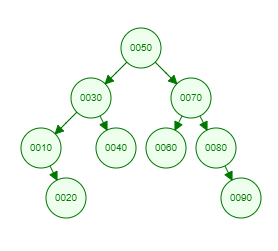Recent Tutorials and Articles
Implementing Spiral Order Traversal of Binary Tree in Java
Published on: 27th October 2018

This tutorial provides code for implementing Spiral order traversal of a Binary Tree.

# Spiral Order Traversal of Binary Tree

Binary Tree traversal is a process of visiting each node of Binary Tree exactly once. Spiral Order traversal falls into Breadth first search category as it moves through all the siblings of a node before moving it to its children.

Spiral Order traversal is similar to level order traversal with only difference that each successive level is traversed in different direction from current level. For example, first level is traversed from left to right, second level from right to left, third level from left to right making it look like covering nodes in a spiral order.

Let's consider below Binary Tree -Here is the Spiral order traversal  result of above tree -

``````50
70 30
10 40 60 80
90 20
``````

# Java Implementation of Spiral Order Traversal of Binary Tree

Here is Java code for Binary Tree containing methods for inserting data and Spiral order traversal -

``````package com.aksain.data.structures;

import java.util.Stack;

public class BinaryTreeWithSpiralOrderTraversal<T extends Comparable<T>> {
private Node root;

private class Node {
T data;
Node left;
Node right;

private Node(T data) {
this.data = data;
}
}

private Node doInsert(T data, Node node) {
if (node == null) {
return new Node(data);
}
int result = data.compareTo(node.data);
if (result < 0) {
node.left = doInsert(data, node.left);
} else if (result > 0) {
node.right = doInsert(data, node.right);
}

return node;
}

public void insert(T data) {
root = doInsert(data, root);
}

private void doSpiralOrderTraversal(Node root) {
final Stack<Node> leftToRight = new Stack<>();

final Stack<Node> rightToLeftQueue = new Stack<>();

while (!leftToRight.isEmpty() || !rightToLeftQueue.isEmpty()) {
while (!leftToRight.isEmpty()) {
Node tmp = leftToRight.pop();
System.out.print(tmp.data + " ");
if (tmp.left != null) {
rightToLeftQueue.push(tmp.left);
}
if (tmp.right != null) {
rightToLeftQueue.push(tmp.right);
}
}
System.out.println();

while (!rightToLeftQueue.isEmpty()) {
Node tmp = rightToLeftQueue.pop();
System.out.print(tmp.data + " ");
if (tmp.right != null) {
leftToRight.push(tmp.right);
}
if (tmp.left != null) {
leftToRight.push(tmp.left);
}
}
System.out.println();
}

}

public void spiralOrderTraversal() {
doSpiralOrderTraversal(root);
}

public static void main(String[] args) {
final BinaryTreeWithSpiralOrderTraversal<Integer> binaryTree = new BinaryTreeWithSpiralOrderTraversal<>();
binaryTree.insert(50);
binaryTree.insert(30);
binaryTree.insert(10);
binaryTree.insert(20);
binaryTree.insert(40);
binaryTree.insert(70);
binaryTree.insert(60);
binaryTree.insert(80);
binaryTree.insert(90);

binaryTree.spiralOrderTraversal();
}

}
``````

Here is the output of above code -

``````50
70 30
10 40 60 80
90 20
``````

Thank you for reading through the tutorial. In case of any feedback/questions/concerns, you can communicate same to us through your comments and we shall get back to you as soon as possible.

Published on: 27th October 2018